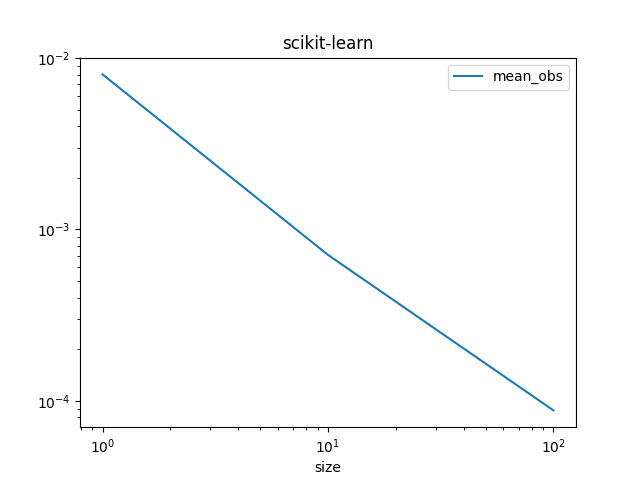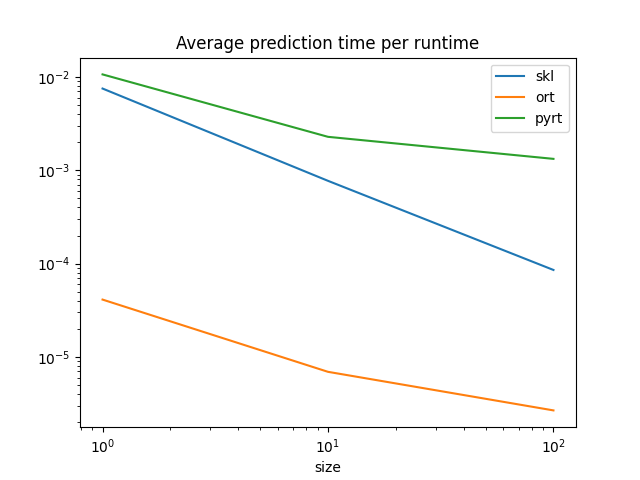# Benchmark ONNX conversion#

Example Train and deploy a scikit-learn pipeline converts a simple model. This example takes a similar example but on random data and compares the processing time required by each option to compute predictions.

## Training a pipeline#

```import numpy
from pandas import DataFrame
from tqdm import tqdm
from onnx.reference import ReferenceEvaluator
from sklearn import config_context
from sklearn.datasets import make_regression
from sklearn.ensemble import (
RandomForestRegressor,
VotingRegressor,
)
from sklearn.linear_model import LinearRegression
from sklearn.model_selection import train_test_split
from onnxruntime import InferenceSession
from skl2onnx import to_onnx
from skl2onnx.tutorial import measure_time

N = 11000
X, y = make_regression(N, n_features=10)
X_train, X_test, y_train, y_test = train_test_split(X, y, train_size=0.01)
print("Train shape", X_train.shape)
print("Test shape", X_test.shape)

reg2 = RandomForestRegressor(random_state=1)
reg3 = LinearRegression()
ereg = VotingRegressor([("gb", reg1), ("rf", reg2), ("lr", reg3)])
ereg.fit(X_train, y_train)
```
```Train shape (110, 10)
Test shape (10890, 10)
```
```VotingRegressor(estimators=[('gb', GradientBoostingRegressor(random_state=1)),
('rf', RandomForestRegressor(random_state=1)),
('lr', LinearRegression())])```
In a Jupyter environment, please rerun this cell to show the HTML representation or trust the notebook.

## Measure the processing time#

We use function `skl2onnx.tutorial.measure_time()`. The page about assume_finite may be useful if you need to optimize the prediction. We measure the processing time per observation whether or not an observation belongs to a batch or is a single one.

```sizes = [(1, 50), (10, 50), (100, 10)]

with config_context(assume_finite=True):
obs = []
for batch_size, repeat in tqdm(sizes):
context = {"ereg": ereg, "X": X_test[:batch_size]}
mt = measure_time(
"ereg.predict(X)", context, div_by_number=True, number=10, repeat=repeat
)
mt["size"] = context["X"].shape
mt["mean_obs"] = mt["average"] / mt["size"]
obs.append(mt)

df_skl = DataFrame(obs)
df_skl
```
```  0%|          | 0/3 [00:00<?, ?it/s]
33%|███▎      | 1/3 [00:07<00:14,  7.06s/it]
67%|██████▋   | 2/3 [00:12<00:06,  6.25s/it]
100%|██████████| 3/3 [00:14<00:00,  4.01s/it]
100%|██████████| 3/3 [00:14<00:00,  4.70s/it]
```
average deviation min_exec max_exec repeat number size mean_obs
0 0.014108 0.004352 0.008928 0.029686 50 10 1 0.014108
1 0.011358 0.003103 0.008228 0.020336 50 10 10 0.001136
2 0.013486 0.002790 0.009885 0.018525 10 10 100 0.000135

Graphe.

```df_skl.set_index("size")[["mean_obs"]].plot(title="scikit-learn", logx=True, logy=True)
```## ONNX runtime#

The same is done with the two ONNX runtime available.

```onx = to_onnx(ereg, X_train[:1].astype(numpy.float32), target_opset=14)
sess = InferenceSession(onx.SerializeToString(), providers=["CPUExecutionProvider"])
oinf = ReferenceEvaluator(onx)

obs = []
for batch_size, repeat in tqdm(sizes):
# scikit-learn
context = {"ereg": ereg, "X": X_test[:batch_size].astype(numpy.float32)}
mt = measure_time(
"ereg.predict(X)", context, div_by_number=True, number=10, repeat=repeat
)
mt["size"] = context["X"].shape
mt["skl"] = mt["average"] / mt["size"]

# onnxruntime
context = {"sess": sess, "X": X_test[:batch_size].astype(numpy.float32)}
mt2 = measure_time(
"sess.run(None, {'X': X})",
context,
div_by_number=True,
number=10,
repeat=repeat,
)
mt["ort"] = mt2["average"] / mt["size"]

# ReferenceEvaluator
context = {"oinf": oinf, "X": X_test[:batch_size].astype(numpy.float32)}
mt2 = measure_time(
"oinf.run(None, {'X': X})",
context,
div_by_number=True,
number=10,
repeat=repeat,
)
mt["pyrt"] = mt2["average"] / mt["size"]

# end
obs.append(mt)

df = DataFrame(obs)
df
```
```  0%|          | 0/3 [00:00<?, ?it/s]
33%|███▎      | 1/3 [00:15<00:31, 15.60s/it]
67%|██████▋   | 2/3 [00:40<00:21, 21.10s/it]
100%|██████████| 3/3 [01:03<00:00, 21.84s/it]
100%|██████████| 3/3 [01:03<00:00, 21.09s/it]
```
average deviation min_exec max_exec repeat number size skl ort pyrt
0 0.012201 0.003428 0.008608 0.021660 50 10 1 0.012201 0.000030 0.018960
1 0.011550 0.003386 0.008357 0.023143 50 10 10 0.001155 0.000021 0.003811
2 0.013936 0.004899 0.009295 0.023858 10 10 100 0.000139 0.000004 0.002129

Graph.

```df.set_index("size")[["skl", "ort", "pyrt"]].plot(
title="Average prediction time per runtime", logx=True, logy=True
)
```ONNX runtimes are much faster than scikit-learn to predict one observation. scikit-learn is optimized for training, for batch prediction. That explains why scikit-learn and ONNX runtimes seem to converge for big batches. They use similar implementation, parallelization and languages (C++, openmp).

Total running time of the script: (1 minutes 19.181 seconds)

Gallery generated by Sphinx-Gallery